# 科技树

2020-03-13更新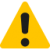Every technology needs a certain amount of science packs per science pack type to be researched, for example the Logistics technology requires 20 automation science packs to be placed in labs while the technology is selected for research.

## 相关成就

• Completing infinite technologies of any level is not required for Tech maniac. All non-infinite levels of technologies that have infinite continuations are still required.

## 无限科技

While most technologies in Factorio are either one-off or have a finite, relatively small number of levels available, a few are "infinite", meaning the player can research as many levels as they can afford. All of them unlock bonuses to existing technologies, never new structures or abilities. The per-level bonuses are constant for a particular infinite technologies and, like finite research bonuses, are additive within a single technology. They are subject to diminishing returns; thus, the per-level and per-science pack contributions from very high levels of infinite technologies will eventually provide only marginal improvements.

All infinite technologies levels require space science packs, and are also the only technologies that do. As such, they are late-game technologies intended primarily for players who wish to continue playing and expand their factory past the nominal victory condition of launching a rocket.

Infinite technologies are identified in-game by a small `∞` infinity symbol shown in the top right corner of the research technology's card in the research screen.

Most infinite technologies are continuations of ordinary multi-level technologies; the "infinite" mechanic becomes effective once the player reaches the card initially labeled with `N - ∞` in the research tree. Only the two artillery-related technologies (artillery shell range and shooting speed) are infinite-only; for these, `1 - ∞` is shown before any levels in them are researched. In either case, once the first infinite level is researched, the card label switches to the one discussed above.

### 定价公式

The price of all infinite technologies is generated in a mathematical progression; for the majority of technologies, the progression is geometric, mostly in powers of 2. Two technologies - mining productivity and follower robot count - use an arithmetic progression instead.

The table below summarizes for all infinite researches their first infinite level, the cost of the first infinite level, the cost formula and the per-level bonus.

We denote by `N` the current level of the research, by `F` the final non-infinite level of the research (hence F+1 is the first "infinite" level) and by `P[N]` the price of the research at level `N`.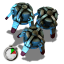Worker robot speed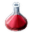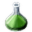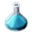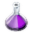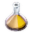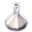7 1,000 1,000 × 2^(N - F - 1) +65% Robot Speed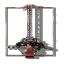Mining productivity4 2,500 2,500 × (N - F - 1) +10% Mining ProductivityPhysical projectile damage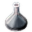7 1,000 1,000 × 2^(N - F - 1) +40% Bullet Damage
+70% Turret Damage
+40% Shotgun Shell Damage
+100% Cannon Shell Damage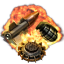Stronger explosives7 1,000 1,000 × 2^(N - F - 1) +50% Rocket Damage
+20% Landmine DamageRefined flammables7 1,000 1,000 × 2^(N - F - 1) +20% Flame Thrower Damage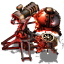Energy weapons damage7 1,000 1,000 × 2^(N - F - 1) +70% Laser Turret Damage
+30% Combat Robot Beam/Laser DamageArtillery shell range1 1,000 1,000 × 2^(N - F) +30% Artillery Shell Range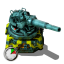Artillery shell speed1 1,000 1,000 + 1,000 × 3^(N - F - 1) +100% Artillery SpeedFollower robot count7 1,000 1,00 × (N - F) + 900 +10 Follower Robots

### 累计成本

As the price of most infinite technologies (specifically, those based on geometric progressions) increases very steeply, it may be a good idea for players to set realistic target levels for each of the infinite technologies they wish to pursue, and make their factory plans accordingly. To that end, the following properties of cumulative infinite research prices may be useful:

1. For infinite technologies whose underlying equation is a powers-of-two geometric series, the cumulative price of the first `N - F` infinite levels (skipping the first `F` level, so counting "infinite" levels only) is `2 × P[N] - P[F+1]`; i.e., twice the price of the final researched level, less the price of the first "infinite" level.
• As N increases, this is approximated well by `2 × P[N] = P[N+1]`, so the cumulative cost of researching to level `N` is about as much as researching level `N+1`.
• If one decides a level `M` which one considers the "highest feasible" with their current science pack production capacity, expanding said capacity by a factor of `X` will allow about `log(X)` additional levels to be researched before the next level takes longer to research with the expanded capacity than level `M + 1` would have taken with the pre-expansion production capacity.
• For example, if one expands production capacity by a factor of 10, they will be able to research at least `floor(log(10)) = 3` and at most `ceiling(log(10)) = 4` additional levels in a given technology before the exponential increase in price negates the speed benefits of their ×10 capacity expansion.
2. The cumulative price of the first `N - F` levels of infinite technologies whose underlying equation is an arithmetic series is `(N - F) × (P[N] + P[F + 1]) ÷ 2`; i.e, `N - F` times the mean of the prices of the first and last "infinite" level. For the Follower robot count (research), an additional `900 × (N-F)` need to be added.
• Expanding production capacity by a factor of `X`, as above, will in this case allow an additional `N × (X - 1)` levels to be researched before the benefit of the expansion is wiped out (i.e., research progress speed drops to or below what it was pre-expansion).
3. The cumulative price of the first N levels of artillery shell shooting speed, the sole infinite technology whose underlying equation is a powers-of-three geometric series (equation type (2)) is `1.5 × P[N] - 0.5 × P`; i.e., 1.5 times the price of the final researched level, less half the price of the first level.

Note that these prices reflect research units, which will not be equal to science packs if productivity modules are used in labs. (In that case, the science pack requirement will be lower.)